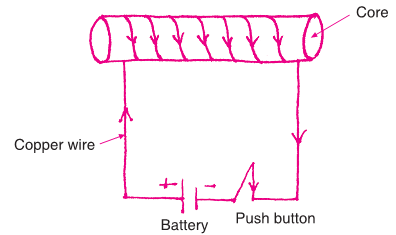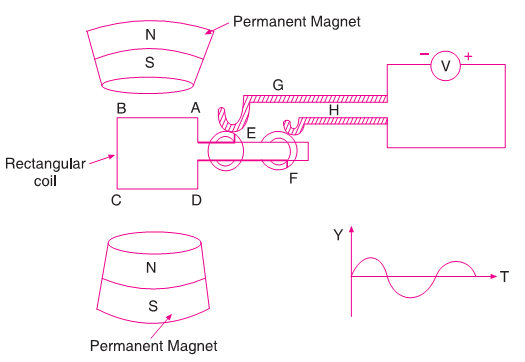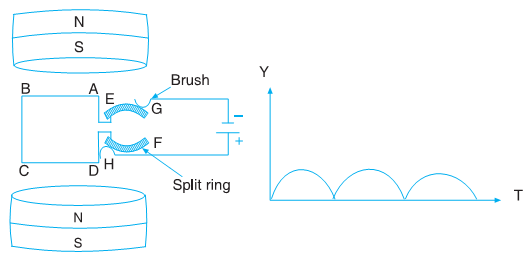# Magnetism

When electric current passes through current carrying conductor or coil then a magnetic field is produced around it. Opposite to this is if a continuous change in magnetic field is produced then electric current can be produced.

### Magnet

A magnet is a material or object that produces a magnetic field which is responsible for a force that pulls or attracts on other materials. The magnets that are commonly available in different shapes are:

• Bar magnet
• Horseshoe magnet
• Cylindrical magnet
• Circular magnet
• Rectangular magnet

If a natural magnet is suspended freely with the help of a string, it always rests in the north-south direction. If the magnet is slightly turned from this direction, it still returns to the same. The end that rests towards the north is named as North Pole while the one which ends at south is named as South Pole. They are represented as N and S.

Properties of Magnets

• Attracts iron towards itself.
• Freely suspended magnet always rests at the north-south direction.
• Like poles repel while unlike poles attract.
• If iron pieces are brought near a strong magnet they also start behaving as magnets.
• The poles of a magnet cannot be separated.

### Magnetic Field

Keep a small magnetic needle near a bar magnet. The magnetic needle rotates and stops in a particular direction only. This shows that a force acts on the magnetic needle that makes it rotate and rest in a particular direction only. This force is called torque.

The region around the magnet where the force on the magnetic needle occurs and the needle stops at a specific direction, is called a magnetic field. The direction of magnetic field is represented by magnetic line of forces.

The direction of magnetic needle changes continuously and it takes the curved path while moving from north to south. This curved path is known as magnetic line of forces. Tangent line draw at any point on magnetic line of force, represent the direction of magnetic field at that point. These magnetic line of forces have following properties:

1. Magnetic line of forces always start from north pole and end at south pole of the magnet.
2. These line of forces never intersect each other.
3. Near the poles magnetic lines are very near to each other which shows that magnetic field at the poles is stronger as compare to other parts.

Earth as Magnet

The Earth acts as a giant magnet with south magnetic pole somewhere in the Arctic and north magnetic pole in Antaractic. The Earth also behaves like a bar magnet. It’s hot liquid centre core contains iron and as it moves, it creates an electric current that cause a magnetic field around the Earth.

The Earth has a north and south magnetic pole. These poles are not same with the geographic north and south poles on a map and tilted at an angle of 11.3 degree with repect to it. Due to this, if a magnetic needle is suspended freely, it rests in the north-south direction and is useful for navigation.

Magnetic Field around Current Carrying Wire

If an electric current is made to flow in a wire, magnetic field produce arround it. When electric current flows through a conductor, magnetic field is produced around the conductor.

### Electromagnet

An electromagnet is a type of magnet in which magnetic field is produced by the flow of elctric current.

For making electromagnet take a piece of paper and give a cylindrical shape. Make several turns of a copper wire over this from one end to the other. This is called solenoid a long thin loop of wire. When the ends of the copper wires are attached to the ends of a battery (+ and –) current starts flowing through the coil which starts functioning as a bar magnet.When the flow of current is stopped from the battery, then, its magnetic property ceases. If the +ve and –ve terminals of the battery are reversed, then the poles of the magnet are also reversed.

 Bar Magnet Electromagnet Permanent magnet; magnetic field remains constant. Temporary magnet; magnetism remains till current flows Magnetic strength cannot be reduced or increased Magnetic strength can be changed by changing current Weak Magnet Strong Magnet Poles do not change Poles depend on direction of current

### Force by Magnetic Field

When an electrical conductor is placed in a magnetic field a force acts upon it. The direction of this force always perpendicular to both direction of current and direction of magnetic field and the direction of the flow of current are both perpendicular to the direction of the magnetic field.

Flemings Left Hand Rule

According to Fleming left hand rule, the direction of force applied to a current carrying wire is perpendicular to both the direction of the current as well as the magnetic field.It means that, stretch the thumb, the first finger and the middle finger in such a manner that they are perpendicular to each other i.e. the angle between the pairs of fingers is 90°. Then. if the first finger shows the direction of the magnetic field and the middle one the direction of current flow, then the thumb shows the direction of force F acting on the current carrying conductor.

### Electromagnetic Induction

Electromagnetic induction is the production of an electric current across a conductor moving through a magnetic field.

### Electric Generator

Electric Generator is such a device that converts mechanical energy to electrical energy. Generators are of two types:

1. A.C. Generator (Alternating Current Generator): This produces current that flows in such a manner that its direction and amplitude changes constantly with time.
2. D.C. Generator (Direct Current Generator): This generator produces current that flows in the same direction in a continuous manner.

### A.C. Generator

A.C. generators operate on the principle of electromagnetic induction. Alternating voltage or current may be generated by rotating a coil in the magnetic field or by rotating a magnetic field with a stationary coil. The value of the voltage or current generated depends on:

• Number of turns in the coil
• Strength of the field
• Speed at which the coil or magnetic field rotates

ABCD is a nonconductor frame on which copper wire has been coiled several times to form a rectangular coil. This coil can freely move between the N-S poles. This rectangular coil is made to rotate between two rings E and F. There are two contact brushes G and H attached to the rings respectively.The rectangular frame ABCD moves between the N-S poles due to mechanical energy. The magnetic field entering into the face ABCD of coil increase from zero to some infinite value and continues to increase till the coil becomes normal to the field. The rate, at which the magnetic field liked with coil charges, is the maximum in the begining and then it decrease continuously. Thus, the induced current in the coil is maximum at time, t = 0 and decrease passing time. When the coil become normal to the field the rate at which magnetic flux of force charges become zero and hence current in the coil is zero.

When the coil further rotates the face of the coil through which magnetic field enters start changing the directing of current reversed. It keeps increasing till the plane of the coil does not become parallel to the magnetic field lines. Thus maximum current flows through the coil at this juncture. If the coil is rotated further, the area in contact with DCBA increase and the rate of change of magnetic field area becomes less.

Thus, the amount of current flowing through the coil decreases. When the coil is perpendicular to the magnetic lines of force then, current becomes zero. Now the north pole of the magnet is reversed. Current starts flowing from its original direction.

### D.C. Generator

This also works like the AC generator. There is just one difference in its structure. There is a half rectangular rings rather than E and F rings as in AC generators.

The rectangular frame rotates and moves from a position parallel to the magnetic field to an upper one. The brush present creates a connection as electric current starts flowing. The current flows in the same direction.